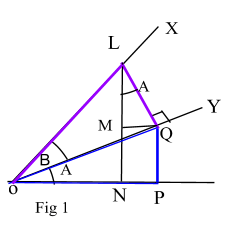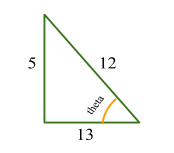# Compound Angle Formulae

Contents

## Summary

### Compound angle formulas are:

•$\sin { (A\quad +\quad B) } \quad =\quad \cos { \quad A\quad \sin { \quad B } } +\quad \sin { \quad A\quad \cos { \quad B } }$
•$\sin { (A\quad -\quad B) } \quad =\quad \sin { \quad A\quad \cos { \quad B\quad -\quad \cos { \quad A\quad \sin { \quad B } } } }$
•$\cos { (A\quad +\quad B) } \quad =\quad \cos { \quad A\quad } \cos { \quad B } \quad -\quad \sin { \quad A } \quad \sin { \quad B }$
•$\cos { (A\quad -\quad B) } \quad =\quad \cos { \quad A\quad } \cos { \quad B } \quad +\quad \sin { \quad A } \quad \sin { \quad B }$
•$\tan { (A\quad -\quad B) } \quad =\quad \frac { \tan { \quad A\quad -\quad \tan { \quad B } } }{ 1\quad +\quad \tan { A\tan { B } \quad \quad } } \quad$
•$\tan { (A\quad +\quad B) } \quad =\quad \frac { \tan { \quad A\quad +\quad \tan { \quad B } } }{ 1\quad -\quad \tan { A\tan { B } \quad \quad } } \quad$

### Half angle formulas are:

•$\sin { (2A) } \quad =\quad 2\quad \cos { A } \quad \sin { A }$
•$\cos { 2A\quad =\quad { cos }^{ 2 } } A\quad -\quad { sin }^{ 2 }A,\quad cos2A\quad =\quad 1\quad -\quad 2{ sin }^{ 2 }A,\quad cos2A\quad =\quad 2{ cos }^{ 2 }A\quad -\quad 1$
•$tan(2A)\quad =\quad \frac { 2tanA }{ 1\quad -\quad { tan }^{ 2 }A }$

### Function to trigonometric form:

•$\alpha \quad cos\theta \quad +\quad b\quad sin\theta \quad =\quad R\quad cos(\theta \quad +\quad \alpha )$

In Fig 1,$P\hat { O } { \underset { \sim }{ O } }\quad =\quad A$ and$L\hat { O } { \underset { \sim }{ O } }\quad =\quad B$ are acute angles and$L\hat { O } { N }\quad =\quad A\quad +\quad B$$L\underset { \sim }{ O } \quad is\quad \bot \quad to\quad OY$$LN\quad is\quad \bot \quad to\quad OP\quad \& \quad \underset { \sim }{ O } P\quad is\quad \bot \quad to\quad OP$$\underset { \sim }{ O } M\quad is\quad \bot \quad to\quad LN$$OP\quad is\quad parallel\quad to\quad M\underset { \sim }{ O }$$Now\quad M\hat { L } \underset { \sim }{ O } \quad =\quad A\quad an\quad L\overset { \wedge }{ \underset { \sim }{ O } } M\quad =\quad 90\quad -\quad A$$sin(A\quad +\quad B)\quad =\quad \frac { LN }{ OL } \quad =\quad \frac { LM\quad +\quad MN }{ OL } \quad =\quad \frac { LM }{ OL } \quad +\quad \frac { MN }{ OL }$

As$MN\quad =\quad \underset { \sim }{ O } P$$=\quad \frac { LM }{ LQ } \quad *\quad \frac { LQ }{ OL } \quad +\quad \frac { QP }{ OQ } \quad *\quad \frac { OQ }{ OL }$$=\quad cosA\quad sinB\quad +\quad sinA\quad cosB$

Hence,$sin(A\quad +\quad B)\quad =\quad cosA\quad sinB\quad +\quad sinA\quad cosB\quad \quad \quad =>\quad i)$

Replacing B by -B in i)$sin(A\quad -\quad B)\quad =\quad sinA\quad cosA\quad -\quad cosA\quad sinB\quad \quad \quad =>\quad ii)$

Also from the diagram:$cos(A\quad +\quad B)\quad =\quad \frac { ON }{ OL } \quad =\quad \frac { OP\quad -\quad PN }{ OL } \quad =\quad \frac { OP }{ OL } \quad -\quad \frac { PN }{ OL }$$=\quad \frac { OP }{ OQ } \quad *\quad \frac { OQ }{ OL } \quad +\quad \frac { PN }{ LQ } \quad *\quad \frac { LQ }{ OL }$

Hence,$cos(A\quad+\quad B)\quad =\quad cosA\quad cosB\quad -\quad sinA\quad sinB\quad \quad \quad =>\quad iii)$$cos(A\quad -\quad B)\quad =\quad cosA\quad cosB\quad +\quad sinA\quad sinB\quad \quad \quad =>\quad iv)$

Also:$tan(A\quad -\quad B)\quad =\quad \frac { tanA\quad -\quad tanB }{ 1\quad +\quad tanA\quad tanB } \quad \quad \quad =>\quad v)$$tan(A\quad +\quad B)\quad =\quad \frac { tanA\quad +\quad tanB }{ 1\quad -\quad tanA\quad tanB } \quad \quad \quad =>\quad vi)$

We have derived the compound angle formulae above.

### All the compound angle formulas are listed below:

•$\sin { (A\quad +\quad B) } \quad =\quad \cos { \quad A\quad \sin { \quad B } } +\quad \sin { \quad A\quad \cos { \quad B } }$
•$\sin { (A\quad -\quad B) } \quad =\quad \sin { \quad A\quad \cos { \quad B\quad -\quad \cos { \quad A\quad \sin { \quad B } } } }$
•$\cos { (A\quad +\quad B) } \quad =\quad \cos { \quad A\quad } \cos { \quad B } \quad -\quad \sin { \quad A } \quad \sin { \quad B }$
•$\cos { (A\quad -\quad B) } \quad =\quad \cos { \quad A\quad } \cos { \quad B } \quad +\quad \sin { \quad A } \quad \sin { \quad B }$
•$\tan { (A\quad -\quad B) } \quad =\quad \frac { \tan { \quad A\quad -\quad \tan { \quad B } } }{ 1\quad +\quad \tan { A\tan { B } \quad \quad } } \quad$
•$\tan { (A\quad +\quad B) } \quad =\quad \frac { \tan { \quad A\quad +\quad \tan { \quad B } } }{ 1\quad -\quad \tan { A\tan { B } \quad \quad } } \quad$

### Double Angle formulae

We use compound angle formulas from above to find double angle formula i.e

If:$sin(A\quad +\quad B)\quad =\quad cosA\quad sinB\quad +\quad sinA\quad cosB$

Replacing B by A:$sin(A\quad +\quad A)\quad =\quad cosA\quad sinA\quad +\quad sinAcosA$$sin(2A)\quad =\quad 2\quad cosA\quad sinA$

Similar replace B by A:$cos(A\quad +\quad B)\quad =\quad cosA\quad cosB\quad -\quad sinA\quad sinB$$cos(A\quad +\quad A)\quad =\quad cosA\quad cosA\quad -\quad sinA\quad sinA$$cos\quad 2A\quad =\quad { cos }^{ 2 }A\quad -\quad { sin }^{ 2 }A$$cos\quad 2A\quad =\quad 1\quad -\quad { 2sin }^{ 2 }A$$cos\quad 2A\quad =\quad 2{ cos }^{ 2 }A\quad -\quad 1$

Finally,$tan(A\quad +\quad A)\quad =\quad \frac { tanA\quad +\quad tanA }{ 1\quad -\quad tanAtanA }$$tan(2A)\quad =\quad \frac { tanA\quad +\quad tanA }{ 1\quad -\quad tanAtanA }$$tan(2A)\quad =\quad \frac { 2tanA }{ 1\quad -\quad { tan }^{ 2 }A }$

#### Example #1

Q. Given that$sin\theta \quad =\quad \frac { 5 }{ 13 }$, where$\theta$ is obtuse, find the exact value of

i) sin 2$\theta$

ii) cos 2$\theta$

iii)tan 2$\theta$

Solution:

As$sin\theta \quad =\quad \frac { 5 }{ 13 }$By pythagoras theorem${ 5 }^{ 2 }\quad +\quad { x }^{ 2 }\quad =\quad { 13 }^{ 2 }$$x\quad =\quad 12$

So, now we know$cos\theta \quad =\quad \frac { -12 }{ 13 }$ and$tan\theta \quad =\quad \frac { -5 }{ 12 }$ (minus sign because theta is in the second quadrant and cos & tan are negative in the second quadrant)

i)$sin\theta \quad =\quad 2sin\theta \quad cos\theta$$=\quad 2(\frac { 5 }{ 13 } )(\frac { -12 }{ 13 } )$

Ans:$sin2\theta \quad =\quad \frac { -120 }{ 169 }$

ii)$cos2\theta \quad =\quad { cos }^{ 2 }\theta \quad -\quad { sin }^{ 2 }\theta$$=\quad { (\frac { -12 }{ 13 } ) }^{ 2 }\quad -\quad { \left( \frac { 5 }{ 13 } \right) }^{ 2 }$

Ans:$cos2\theta \quad =\quad \frac { 119 }{ 169 }$

iii)$tan2\theta \quad =\quad \frac { 2tan\theta }{ 1\quad -\quad { tan }^{ 2 }\theta }$$=\quad \frac { 2tan(-{ 5 }/{ 12 }) }{ 1\quad -\quad { tan }^{ 2 }(-{ 5 }/{ 12 }) }$

Ans:$tan2\theta \quad =\quad \frac { -120 }{ 119 }$

Function$\alpha \quad cos\quad \theta \quad +\quad b\quad sin\quad \theta$

Suppose a function$f\left( \theta \right) \quad =\quad \alpha \quad cos\quad \theta \quad +\quad b\quad sin\quad \theta$ , where a and b are constants, it can be easily converted into a single trigonometric function of the form$R\quad cos(\theta \quad +\quad \alpha )$ where R is a positive constant and$\alpha$ is an acute angle.

#### Example #2

If we have a function:$f\left( \theta \right) \quad =\quad 7\quad cos\quad \theta \quad +\quad 24\quad sin\quad \theta$

We equate it with its trigonometric form:$7\quad cos\quad \theta \quad +\quad 24\quad sin\quad \theta \quad =\quad R\quad cos(\theta \quad +\quad \alpha )$

Expand the right hand side:$7\quad cos\quad \theta \quad +\quad 24\quad sin\quad \theta \quad =\quad R\quad cos\theta cos\alpha \quad -\quad Rsin\theta sin\alpha \quad$

Compare the coefficients of$cos\theta$ and$sin\theta$:$7\quad =\quad R\quad cos\alpha$$24\quad =\quad R\quad sin\alpha$

To find R we take the magnitude of 7 and 24$R\quad =\quad \sqrt { { (7) }^{ 2 }\quad +\quad { (24) }^{ 2 } }$$R\quad =\quad 25$

To find$\alpha$ we do:$tan(\alpha )\quad =\quad \frac { 27 }{ 7 }$$\alpha \quad =\quad 73.7$°

Ans: Hence the trigonometric form is$25cos(\theta \quad +\quad 73.7$°$)$